### Home > PC > Chapter 9 > Lesson 9.2.1 > Problem9-70

9-70.
1. Let s(x) = sin x. Homework Help ✎

1. Find the exact value of the average rate of change of s(x) from x =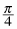to x =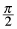.

2. Write the expression for the average rate of change of s(x) from x =to x =+ h.

3. Use the fact that sin(A + B) = sin A cos B + cos A sin B to show that the expression in part (b) can be written as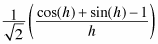.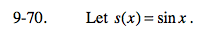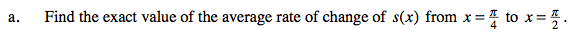$\frac{s\left(\frac{\pi}{2}\right)-s\left(\frac{\pi}{4}\right)}{\left(\frac{\pi}{2}\right)-\left(\frac{\pi}{4}\right)}$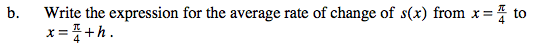$\frac{s\left(\frac{\pi}{4}+h\right)-s\left(\frac{\pi}{4}\right)}{\left(\frac{\pi}{4}+h\right)-\left(\frac{\pi}{4}\right)}$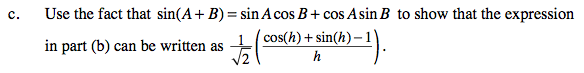$m=\frac{\text{sin}\left ( \frac{\pi }{4}+h \right )-\text{sin}\left ( \frac{\pi }{4} \right )}{h}=\frac{\text{sin}\left ( \frac{\pi }{4}\right )\text{cos}(h)+\cos\left ( \frac{\pi }{4} \right )\text{sin}(h)-\text{sin}\left ( \frac{\pi }{4} \right )}{h}$

Substitute values for the trigonometric expressions and simplify.